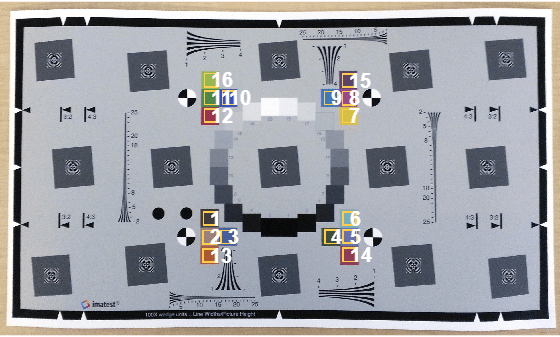# Correct Colors Using Color Correction Matrix

This example shows how to adjust the colors of an image to better match a standardized set of colors on an Imatest® edge spatial frequency response (eSFR) test chart.

### Obtain Color Correction Matrix from the Test Chart Image

Read an image of a test chart, and create a copy of the image in the linear RGB color space.

```I = imread("eSFRTestImage.jpg"); Ilin = rgb2lin(I);```

Create an `esfrChart` object that stores information about the test chart. Display the chart, highlighting the 16 color patches. The image has a blue tint.

```chart = esfrChart(I); displayChart(chart,displayEdgeROIs=false, ... displayGrayROIs=false,displayRegistrationPoints=false)```Measure the color accuracy of the 16 color patches by using the `measureColor` function. The function also returns the color correction matrix that is used to perform the color correction.

`[colorTable,ccm] = measureColor(chart);`

Compare the measured and reference colors on a color patch diagram. The closer the `Delta_E` value is to 1, the less perceptible the color difference is.

`displayColorPatch(colorTable)`### Color-Correct the Test Chart Image

Color-correct the original test chart image in the linear RGB color space.

`Ilin_corrected = imapplymatrix(ccm(1:3,:)',Ilin,ccm(4,:));`

Convert the color-corrected image to the sRGB color space and display the result.

```I_corrected = lin2rgb(Ilin_corrected); imshow(I_corrected) title("Color-Corrected Image Using Color Patches")```Create an `esfrChart` object that stores information about the color-corrected test chart. Measure the color accuracy of the 16 color-corrected color patches in the sRGB color space.

```chart_corrected = esfrChart(I_corrected); colorTable_corrected = measureColor(chart_corrected);```

Compare the corrected and reference colors on a color patch diagram. The measured color errors, `delta_E`, are smaller for the color-corrected image than for the original image. Therefore, the colors in this image better agree with the reference colors. However, the chart now has an overall yellow tint and the contrast of the image has decreased.

`displayColorPatch(colorTable_corrected)`### Improve Color Correction Using Gray Patches

You can improve the color correction by including the gray patches as well as the color patches in the least squares fit. Display the original chart, highlighting the 20 gray patches and 16 color patches.

```displayChart(chart,displayEdgeROIs=false, ... displayRegistrationPoints=false)```Get the reference L*a*b* values of the color and grayscale patches, which are stored in the `ReferenceColorLab` and `ReferenceGrayLab` properties of the eSFR chart object. Convert these values from the sRGB color space to the linear RGB color space.

```referenceLab = [chart.ReferenceColorLab; chart.ReferenceGrayLab]; referenceRGB = lab2rgb(referenceLab,outputtype="uint8",ColorSpace="linear-rgb");```

Measure the mean gray value on each of the 20 gray patches in the sRGB color space by using the `measureNoise` function.

```noiseTable = measureNoise(chart); measuredGrayRGB = [noiseTable.MeanIntensity_R, ... noiseTable.MeanIntensity_G, ... noiseTable.MeanIntensity_B];```

Concatenate all measured sRGB color values of the color and grayscale patches, then convert the color values to the linear RGB color space.

```measuredColorRGB = [colorTable.Measured_R, ... colorTable.Measured_G, ... colorTable.Measured_B]; measuredRGB = [measuredColorRGB; measuredGrayRGB]; measuredRGB = rgb2lin(measuredRGB);```

Calculate the color correction matrix.

`ccmWithGray = double([measuredRGB ones(36,1)]) \ double(referenceRGB);`

Perform the color correction and display the result. The chart no longer has a yellow tint and the overall appearance of the chart has improved.

```Ilin_correctedWithGray = imapplymatrix(ccmWithGray(1:3,:)',Ilin,ccmWithGray(4,:)'); I_correctedWithGray = lin2rgb(Ilin_correctedWithGray); imshow(I_correctedWithGray) title("Color-Corrected Image Using Gray and Color Patches")```Compare the corrected and reference colors on a color patch diagram. Some of the measured color errors have decreased, while others have increased.

```chart_correctedWithGray = esfrChart(I_correctedWithGray); colorTable_correctedWithGray = measureColor(chart_correctedWithGray); displayColorPatch(colorTable_correctedWithGray)```